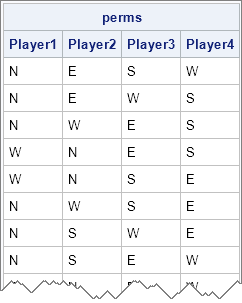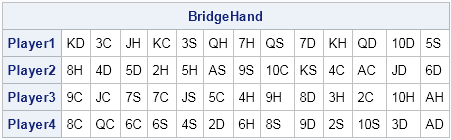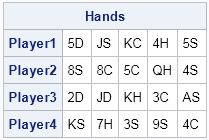I've written several articles that show how to generate permutations in SAS. In the SAS DATA step, you can use the ALLPEM subroutine to generate all permutations of a DATA step array that contain a small number (18 or fewer) elements. In addition, the PLAN procedure enables you to generate permutations that are useful for experimental design.

I also wrote about how to generate random permutations in the SAS/IML language. The random-permutation algorithm also works in the DATA step, but alternatively you can call the RANPERM subroutine in the DATA step to generate a random permutation of elements in an array.

However, I wrote those articles back in 2010, and a lot has changed since then in the SAS/IML language. Beginning in SAS 9.3, the SAS/IML language supports the following functions for generating permutations:

• The ALLPERM function generates all n! permutations of a set with n elements, for n ≤ 18
• The RANPERM function generates random permutations of a set.
• The RANPERK function generates a permutation of k elements chosen from a set that contains n elements.

The RANPERM and RANPERK functions can be used for algorithms in SAS that require random sampling without replacement. (Random sampling without replacement is also supported by the SURVEYSELECT procedure. See the documentation of the METHOD=SRS option.)

### Generating all permutations of N elements

For small sets, the ALLPERM function returns a vector that contains all n! permutations of the elements of the set. Each row is a permutation. For example, what are all the possible arrangements of four players at a card table? (The positions around the table are traditionally labeled North, East, South, and West.) The following SAS/IML statements display all the possible arrangements of players at the four positions:

```proc iml; call randseed(1234); pName = "Player1":"Player4"; perms = allperm({N E S W}); /* use North, East, South, and West as labels */ print perms[c=pName];```For an application that is more statistically oriented, you can use the complete list of permutations to construct an exact permutation test (see documentation for the FREQ procedure).

### Generating random permutations of N elements

Often the complete list of permutations of N elements is too large to compute. In that case, you can often use random permutations to simulate draws from the set of complete permutations. Random permutations are also important because they represent a random rearrangement (a shuffle) of elements.

For example, suppose that you have a deck of 52 playing cards. You can simulate a shuffle of the deck by generating a random permutation of the 52 cards, as follows:
```/* function to generate a deck of 52 playing cards: 2C, 3C,..., KS, AS */ start MakeCardDeck(); suits = {H D C S}; suits52 = rowvec( repeat(suits`,1,13) ); vals = char(2:10,2) || {J Q K A}; vals52 = right( repeat( vals, 1, 4) ); return( vals52 + suits52 ); finish; Cards = MakeCardDeck();   /* randomly permute columns (or rows) of a matrix */ shuffle = ranperm(Cards); BridgeHand = shape(shuffle, 0, 4); print BridgeHand[c=("Player1":"Player4")];```The previous SAS/IML statements create a 52-element character vector to represent to suit and face value of the cards. The array is then randomly permuted. The cards are then "dealt" to four players, who each receive 13 cards. Notice that enumerating all 8.06 x 1067 permutations of 52 elements is infeasible.

### Generating random permutations of k elements from a set of N elements

Sometimes you don't need the entire permutation, but just k elements, which you can think of as the first k elements of the permutation. If that is the case, you don't need to generate the entire permutation. Instead, use the RANPERK function. For example, the following statements return 20 cards (four five-card hands) randomly drawn from a deck (without replacement):

```NumPlayers = 4; NumCards = 5; Deal = ranperk(Cards, NumCards*NumPlayers); Hands = shape(Deal, 0, NumPlayers); print Hands[c=("Player1":"Player4")];```Besides being useful, I like the name of the RANPERK function. A "perk" (slang for perquisite) is an additional benefit given to someone, like free coffee and snacks in the break room. The thought of the SAS/IML language giving me random perks makes me smile!

In the field of statistical sampling, a permutation is a "random sample drawn without replacement" from a finite set where there is an equal probability of choosing any item. The RANPERK function represents drawing k items without replacement from a box that contains N items. The RANPERM function represents drawing without replacement until the box is empty. Thus you can use these two SAS/IML functions to support random sampling without replacement for items that are drawn wih equal probability.

ShareDistinguished Researcher in Computational Statistics

Rick Wicklin, PhD, is a distinguished researcher in computational statistics at SAS and is a principal developer of SAS/IML software. His areas of expertise include computational statistics, simulation, statistical graphics, and modern methods in statistical data analysis. Rick is author of the books Statistical Programming with SAS/IML Software and Simulating Data with SAS.

1.could you please provide an example of code to create a dataset of a total of 25 possible elements for 6 groups with order sensitive and repetition allowed. I am trying to generate basically 25 pricing combinations for each group. this will generate approximately 244,140,625 records. will sas be able to create a dataset this big? thank you in advance!

•I don't understand your questions. Post it with a small example to a SAS Support Community.

2.I have 5 products and 5 different pricing. Say product 1,2,3,4,5 and prices for \$1,\$2,\$3,\$4,\$5. I need to get all combinations between the product and the prices which then gives me 25 possible values (5x5). Now I have 6 groups that I will offer these 25 combinations. I need to generate all possible permutations for these 6 groups allowing repetitions and no blanks.
for example I had set the following definitions for the product and pricing combinations as follows:
a -> product 1 with \$1 fee
b -> product 1 with \$2 fee
.
.
.
f -> product 2 with \$1 fee
g -> product 2 with \$2 fee
.
.
.
In the end I will have 25 product*price combinations that will be represented by the letters "a" through "y"
I have 6 groups in my population that I will be setting offers for and would need to generate a dataset that contain all the possible offers (a thru y) for each group.

example:
group1 group2 group3 group4 group5 group6
a a a a a a
a a a a a b
a a a a a c
.
.
.
a a a a b a
a a a a c a
.
.
.
z z z z z a
z z z z z b
and so on and so forth.

I have seen different coding for permutation but cannot seem to accomodate the total volume of the permutations.

Hope I was able to explain it better this time.
Thank you!

•The explanation is fine. Post it to a SAS Support Community.